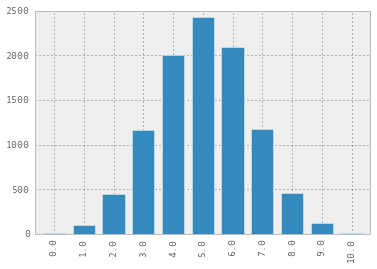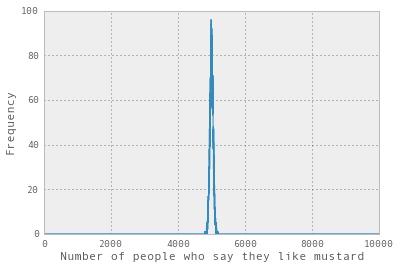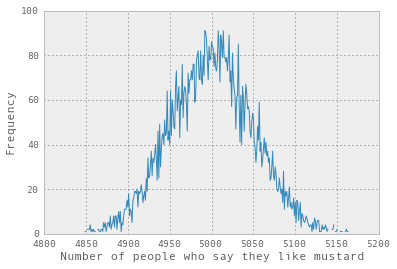# Fun with stats: How big of a sample size do I need?

[There’s a version of this post with calculations on nbviewer!]

“How do I decide how big of a sample size I need for an experiment?”

## Flipping a coin

I’ll do my best to answer, but first let’s do an experiment! Let’s flip a coin ten times.

> flip_coin(10)
tails    3


Oh man! 70% were heads! That’s a big difference.

NOPE. This was a random result! 10 as a sample size is way too small to decide that. What about 20?

> flip_coin(20)
tails     7


65% were heads! That is still a pretty big difference! NOPE. What about 10000?

> flip_coin(10000)
tails    4982


That’s very close to 50%.

So what we’ve learned already, without even doing any statistics, is that if you’re doing an experiment with two possible outcomes, and you’re doing 10 trials, that’s terrible. If you do 10,000 trials, that’s pretty good, and if you see a big difference, like 80% / 20%, you can almost certainly rely on it.

But if you’re trying to detect a small difference like 50.3% / 49.7%, that’s not a big enough difference to detect with only 10,000 trials.

So far this has all been totally handwavy. There are a couple of ways to formalize our claims about sample size. One really common way is by doing hypothesis testing. So let’s do that!

Let’s imagine that our experiment is that we’re asking people whether they like mustard or not. We need to make a decision now about our experiment.

Step 1: make a null hypothesis

Let’s say that we’ve talked to 10 people, and 710 of them like mustard. We are not fooled by small sample sizes and we ALREADY KNOW that we can’t trust this information. But your brother is arguing “710 seems like a lot! I like mustard! I totally believe this!”. You need to argue with him with MATH.

So we’re going to make what’s called a “null hypothesis”, and try to disprove it. In this case, let’s make the null hypothesis “there’s a 5050 chance that a given person likes mustard”.

So! What’s the probability of seeing an outcome like 710 if the null hypothesis is true? We could calculate this, but we have a computer and I think it’s more fun to use the computer.

So let’s pretend we ran this experiment 10,000 times, and the null hypothesis was true. We’d expect to sometimes get 1010 mustard likers, sometimes 0/10, but mostly something in between. Since we can program, let’s run the asking-10-people experiment 10,000 times!

I programmed it, and here are the results:

0        7
1      102
2      444
3     1158
4     2002
5     2425
6     2094
7     1176
8      454
9      127
10      11


Or, on a pretty graph:Okay, amazing. The next step is:

Step 2: Find out the probability of seeing an outcome this unlikely or more if the null hypothesis is true

The “this unlikely or more” part is key: we don’t want to know the probability of seeing exactly 710 mustard-likers, we want to know the probability of seeing 710 or 810 or 910 or 1010.

So if we add up all the times when 710 or more people liked mustard by looking at our table, that’s about 1700 times, or 17% of the time.

We could also calculate the exact probabilities, but this is pretty close so we won’t. The way this kind of hypothesis testing works is that you only reject the null hypothesis if the probability of seeing this data if it’s true is really low. So here the probability of seeing this data if the null hypothesis is true is 17%. 17% is pretty high, (16!), so we won’t reject it. This value (0.17) is called a p-value by statisticians. We won’t say that word again here though. Usually you want this to be more like 1% or 5%.

We’ve really quickly arrived at

Step 3: Decide whether or not to reject the null hypothesis

If we see that 710 people like mustard, we can’t reject it! If we’d instead seen that 1010 of our survey respondants liked mustard, that would be a totally different story! The probability of seeing that is only about 1010000, or 0.1%. So it would be actually very reasonable to reject the null hypothesis.

### What if we’d used a bigger sample size?

So asking 10 people wasn’t good enough. What if we asked 10,000 people? Well, we have a computer, so we can simulate that!

Let’s flip a coin 10,000 times and count the number of heads. We’ll get a number (like 5,001). Then we’ll repeat that experiment 10,000 times and graph the results. This is like running 10,000 surveys of 10,000 people each.That’s pretty narrow, so let’s zoom in to see better.So in this graph we ran 10,000 surveys of 10,000 people, and in about 100 of them 5000 people said they liked mustard

There are two neat things about this graph. The first neat thing is that it looks like a normal distribution, or “bell curve”. That’s not a coincidence! It’s because of the central limit theorem! MATH IS AMAZING.

The second is how tightly centred it is around 5,000. You can see that the probability of seeing more than 52% or less than 48% is really low. This is because we’ve done a lot of samples.

This also helps us understand how people could have calculated these probabilities back when we did not have computers but still needed to do statistics – if you know that your distribution is going to be approximately the normal distribution (because of the central limit theorem), you can use normal distribution tables to do your calculations.

In this case, “the number of heads you get when flipping a coin 10,000 times” is approximately normally distributed, with mean 5000.

### So how big of a sample size do I need?

Here’s a way to think about it:

1. Pick a null hypothesis (people are equally likely to like mustard or not)
2. Pick a sample size (10000)
3. Pick a test (do at least 5200 people say they like mustard?)
4. What would the probability of your test passing be if the null hypothesis was true? (less than 1%!)
5. If that probability is low, it means that you can reject your null hypothesis! And your less-mathematically-savvy brother is wrong, and you have PROOF.

Some things that we didn’t discuss here, but could have:

• independence (we’re implicitly assuming all the samples are independent)
• trying to prove an alternate hypothesis as well as trying to disprove the null hypothesis

I was also going to do a Bayesian analysis of this same data but I’m going to go biking instead. That will have to wait for another day. Later!

(Thanks very much to the fantastic Alyssa Frazee for proofreading this and fixing my terrible stats mistakes. And Kamal for making it much more understandable. Any remaining mistakes are mine.)